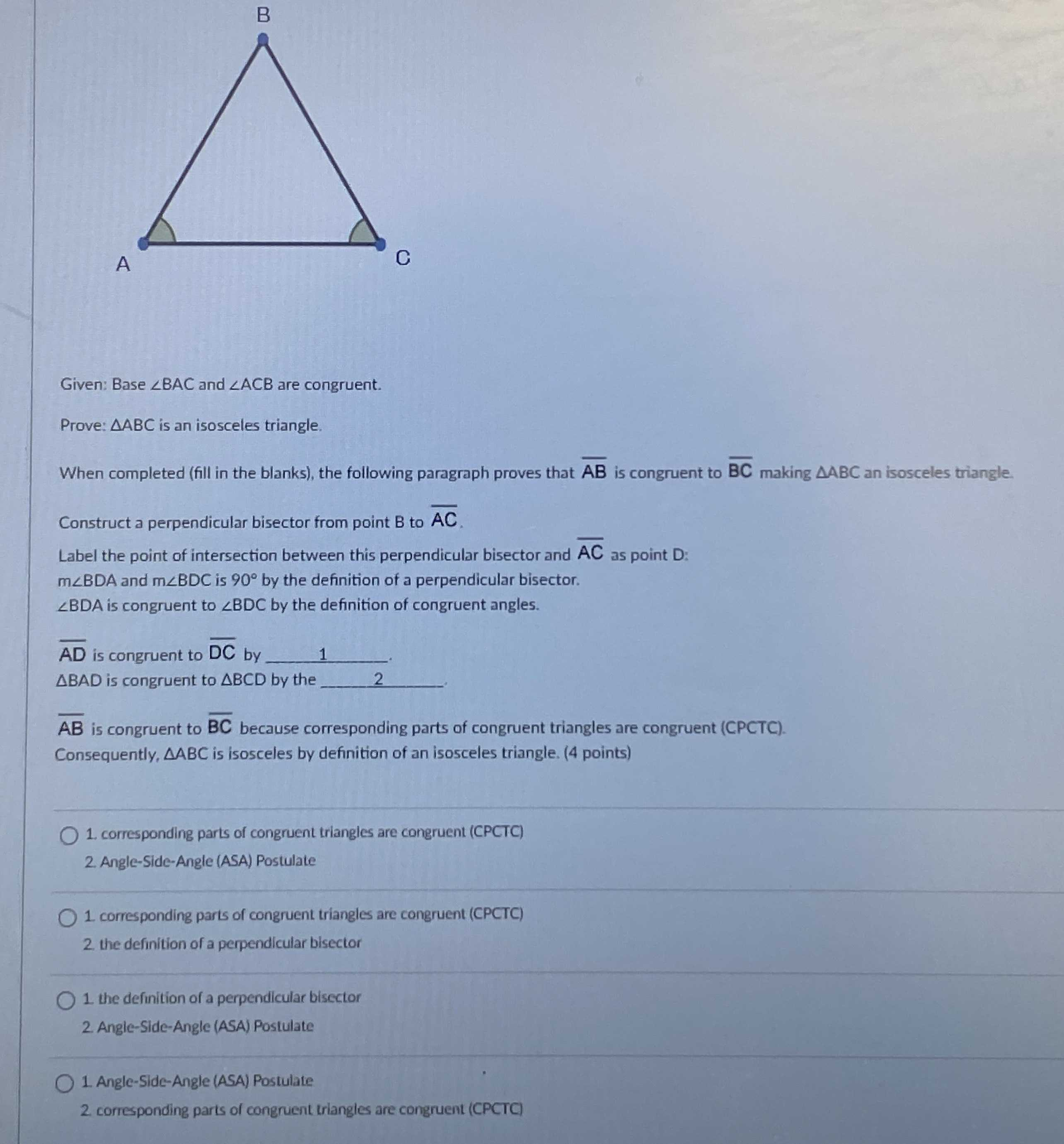### Still have math questions?Given: Base $$\angle B A C$$ and $$\angle A C B$$ are congruent. Prove: $$\triangle A B C$$ is an isosceles triangle. When completed (fill in the blanks), the following paragraph proves that $$\overline { AB }$$ is congruent to $$\overline { BC }$$ making $$\triangle ABC$$ an isosceles triangle. Construct a perpendicular bisector from point $$B$$ to $$\overline { AC }$$ .